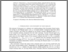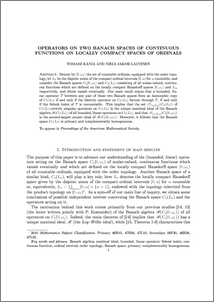# Operators on two Banach spaces of continuous functions on locally compact spaces of ordinals

Kania, Tomasz and Laustsen, Niels (2015) Operators on two Banach spaces of continuous functions on locally compact spaces of ordinals. Proceedings of the American Mathematical Society, 143. pp. 2585-2596. ISSN 0002-9939Preview
PDF (CoLoMaxideal)
CoLoMaxideal.pdf - Accepted Version

## Abstract

Denote by $[0,\omega_1)$ the set of countable ordinals, equipped with the order topology, let $L_0$ be the disjoint union of the compact ordinal intervals $[0,\alpha]$ for $\alpha$ countable, and consider the Banach spaces $C_0[0,\omega_1)$ and $C_0(L_0)$ consisting of all scalar-valued, continuous functions which are defined on the locally compact Hausdorff spaces $[0,\omega_1)$ and $L_0$, respectively, and which vanish eventually. Our main result states that a bounded, linear operator $T$ between any pair of these two Banach spaces fixes an isomorphic copy of $C_0(L_0)$ if and only if the identity operator on $C_0(L_0)$ factors through $T$, if and only if the Szlenk index of $T$ is uncountable. This implies that the set $\mathscr{S}_{C_0(L_0)}(C_0(L_0))$ of $C_0(L_0)$-strictly singular operators on $C_0(L_0)$ is the unique maximal ideal of the Banach algebra $\mathscr{B}(C_0(L_0))$ of all bounded, linear operators on $C_0(L_0)$, and that $\mathscr {S}_{C_0(L_0)}(C_0[0,\omega_1))$ is the second-largest proper ideal of $\mathscr{B}(C_0[0,\omega _1))$. Moreover, it follows that the Banach space $C_0(L_0)$ is primary and complementably homogeneous. - See more at: http://www.ams.org/journals/proc/0000-000-00/S0002-9939-2015-12480-X/home.html#sthash.nZwAr45z.dpuf

Item Type:
Journal Article
Journal or Publication Title:
Proceedings of the American Mathematical Society
First published in Proceedings of the American Mathematical Society in Vol. 143, (2015), published by the American Mathematical Society
Uncontrolled Keywords:
/dk/atira/pure/subjectarea/asjc/2600
Subjects:
Departments:
ID Code:
73313
Deposited By:
Deposited On:
17 Mar 2015 10:44
Refereed?:
Yes
Published?:
Published